# Numericals on Electrostatics

In this page we have Numericals on Electrostatics(Electric Charge,Field & Gauss Laws) for JEE advanced , Main & NEET . Hope you like them and do not forget to like , social share and comment at the end of the page.

Question 1
Two point charges q1 and q2 are located with points having position vectors r1 and r2 .
1. Find the position vector r3 where the third charge q3 should be placed so that force acting on each of the three charges would be equal to zero.
2. Find the amount of charge q3

To solve the problem we choose Cartesian co-ordinates system where $\mathbf{r_1}$ , $\mathbf{r_2}$ and $\mathbf{r_3}$ are position vectors of charge $q_1$, $q_2$ and $q_3$ respectively with respect to the origin O as shown below in the figure.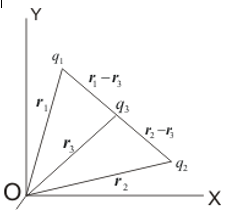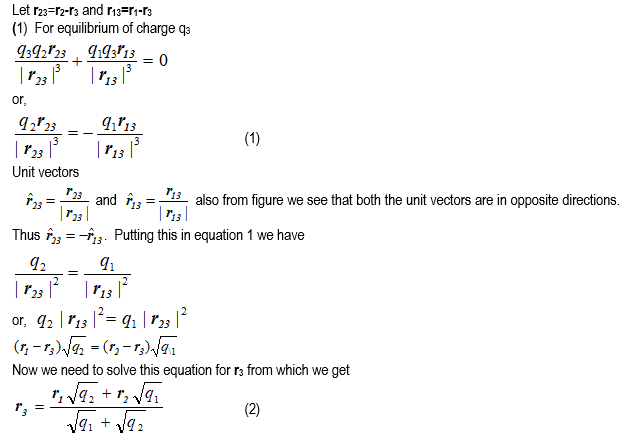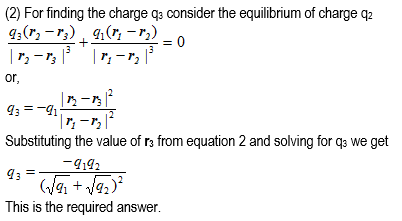Question 2
Consider a thin wire ring of radius R and carrying uniform charge density λ per unit length.
1. Find the magnitude of electric field strength on the axis of the ring as a function of distance x from its centre.
2. What would be the form of electric field function for x>>R.
3. Find the magnitude of maximum strength of electric field.

Consider the figure given below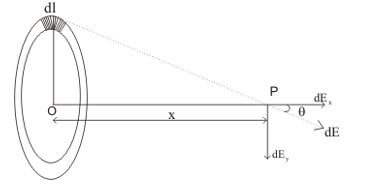Thus, from symmetry considerations total field along Y-axis is zero. Hence,
$E_Y=0$ and $E=E_X$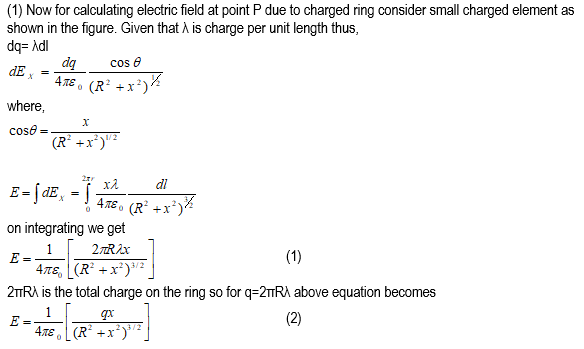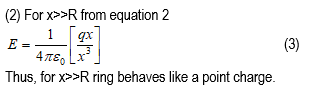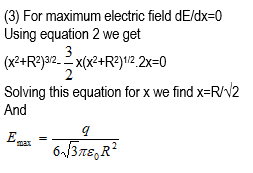Question 3
Two equally charged metal balls each of mass m Kg are suspended from the same point by two insulated threads of length l m long. At equilibrium, as a result of mutual separation between balls, balls are separated by x m. Determine the charge on each ball.

Let Q be the charge on the each of the metal balls A and B suspended from a point O as shown below in the figure. Let r be the distance between two metal balls when they are at equilibrium.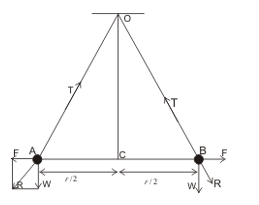Forces acting on the metal ball are
(1) Weight of the metal ball
W= mg
Acting in vertically downward direction.
(2) Force of electrostatic repulsion
$F= \frac {1}{4 \pi \epsilon _0} \frac {Q^2}{r^2}$
directed away from each other.
(3) Tension T in each of the insulated threads directed towards O. Since each of the metal ball is in equilibrium under these three forces, the tension must be equal and opposite to the resultant R of F and W. Considering similar triangles AWR and AOC we have
$\frac {W}{OC} = \frac {F}{CA} = \frac {T}{AO}$
or
$F= W \frac {CA}{OC}$
CA=r/2 and $OC \approx OA=l$ m
Thus
$F= W \frac {r/2}{l}$
Putting the value of F and W we get
$\frac {1}{4 \pi \epsilon _0} \frac {Q^2}{r^2}= \frac {mgr}{2l}$
or
$Q = \sqrt {\frac {2 \pi \epsilon _0 mg r^3}{l}}$

Question 4
There are two identical particles each of mass m and carrying charge Q. Initially one of them is at rest and another charge moves with velocity v directly towards the particle at rest. Find the distance of closest approach.

Given that v is the initial velocity of first charged particle. Due to the repulsion second particle also moves. The velocity of particle moving initially decreases and that of second particle increases. At distance of closest approach velocity of both the particles become equal. From the law of conservation of momentum,
$mv+0=mv^{'}+mv^{'}$
or, $v^{'}=\frac {v}{2}$
Let r be the distance between charged particles at the distance of closest approach then potential energy between them is
$U= \frac {1}{4 \pi \epsilon _0} \frac {Q^2}{r}$
From law of conservation of energy we have
$\frac {1}{2} mv^2 = \frac {1}{4 \pi \epsilon _0} \frac {Q^2}{r} + \frac {1}{2} mv_{'}^2 + \frac {1}{2} mv_{'}^2$
$\frac {1}{4} mv^2= \frac {1}{4 \pi \epsilon _0} \frac {Q^2}{r}$
$r = \frac {1}{4 \pi \epsilon _0} \frac {4Q^2}{mv^2}$

Question 5
Find the electric field at the centre of uniformly charged semi circular arc having linear charge density λ.

Consider the figure given below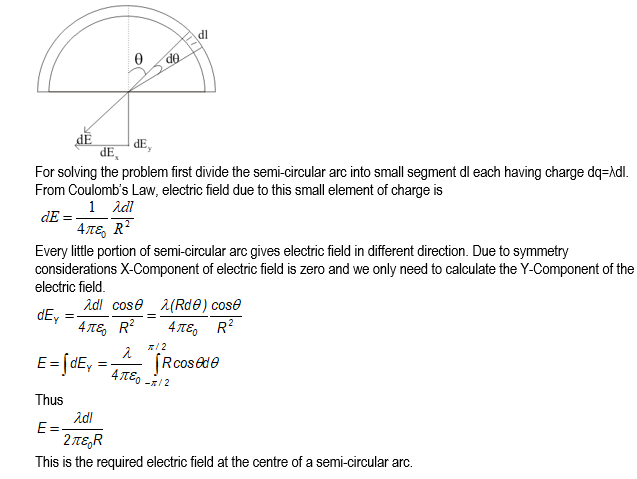Question 6
Two opposite corners of square carry charge –q and other tow opposite corners of same square carry charge +q as shown below in the figure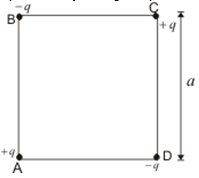All the four charges are equal in magnitude. Find the magnitude and direction of force on the charge on the upper right corner by the other three charges.

The forces acting on the charge +q at point C are $F_A$, $F_B$, and $F_D$ by the charges at corners A, B, and D respectively. Magnitude of force $F_B$ and $F_D$ is
$F_B=F_D= \frac {1}{4 \pi \epsilon _0} \frac {q^2}{a^2}$
and that of $F_A$ is
$F_A= \frac {1}{4 \pi \epsilon _0} \frac {q^2}{(\sqrt{2} a)^2}=\frac {1}{4 \pi \epsilon _0} \frac {q^2}{2a^2}$
$F_B$ and $F_D$ can be regarded as the sum of vector along diagonal CA and one normal to it as shown below in the figure.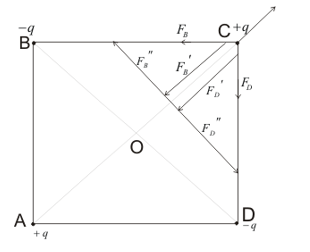Now
$F_D^{"} + F_B^{"}=0$
Thus,
$F_B+ F_D = F_B^{'} + F_D^{'}=2 F_B \cos 45=\frac {\sqrt {2} q^2}{ 4 \pi \epsilon _0 a^2}$
Having direction from C to A. This force is larger then $F_A$. Hence resultant force on charge +q at C is directed from C to A and has magnitude
$F_{res}= ( \sqrt{2} - \frac {1}{\sqrt {2}}) \frac {q^2}{4 \pi \epsilon _0 a^2}$

Question 7
A rigid insulated wire frame in form of a right angled triangle ABC is set in vertical plane as shown below in the figure.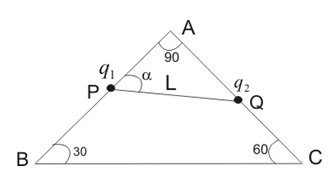Two beads of equal masses m and each carrying charges q1 and q2 are connected by a chord of length l and can slide without friction on the wires. Considering the case when beads are stationary determine
1. the normal reaction on the beads
2. the angle α
3. tension in the chord
If the chord is now cut what are the value of charges for which beads continue to remain stationary.

To solve the problem, we would first have to consider the forces acting on the bead P. Figure below shows the diagrammatic representation of force acting on the bead P.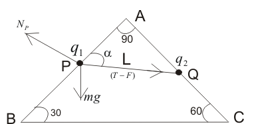Thus, forces acting on bead P are
(i) W=mg, weight of bead acting in vertically downward direction.
(ii) Tension T in the string
(iii) $F=\frac {1}{4 \pi \epsilon _0} \frac {q_1 q_2}{l^2}$ electric force between two beads
(iv) Force of normal reaction.
(T-F) is the net force acting along the string. Bead P would be in equilibrium if net force acting on the bead is zero. We now resolve the components of mg and (T-F) for bead P as shown below in the figure.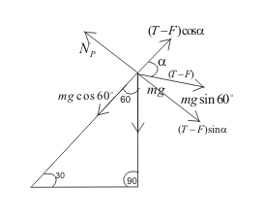Because of equilibrium of charge $q_1$
$N_P=mg \sin {60}^0+\left(T-F \right) \sin {\alpha}$ -(1)
$(T-F) \cos {\alpha}=mg \cos {60}^0$ -(2)
We now resolve the components of mg and (T-F) for bead Q as shown below in the figure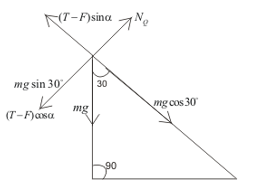Because of equilibrium of charge $q_2$

$N_Q=mg \sin {30}^0+ (T-F) \cos {\alpha}$ --(3)
$(T-F) \sin{\alpha}=mgcos{30}^0$ --(4)
(A) From 1 and 3
$N_P=mg \sin{60}^0+ mg \cos{30}^0= \sqrt{3} mg$
from 2 and 4
$N_Q= mg \sin {30}^0+ mg \cos{60}^0=mg$
This is the required normal reaction on the bead.
(B) dividing equation 4 by 3 we get
$\tan \alpha= \frac {\cos 30^0}{\cos 60^0}=\sqrt {3}= \tan 60^0$
thus $\alpha=60^0$

(C) using $\alpha=60^0$ and $N_Q$ in equation 3
$mg=mg \sin {30}^0 + (T-F) \cos{60}^0$
$mg (1- \sin{30}^0)= (T-F)cos{60}^0$
solving it for T and using $F= \frac {1}{4 \pi \epsilon _0} \frac {q_1 q_2}{l^2}$ , we get
$T=mg + \frac {1}{4 \pi \epsilon_0} \frac{q_1q_2}{l^2}$ --(5)
This is the tension on the chord.
When the chord is cut T=0 thus from equation 5 we have
$mg= -\frac{1}{4 \pi \epsilon_0}\frac{q_1q_2}{l^2}$
The right hand side of this equation should be positive which is possible if charges $q_1$ and $q_2$ have opposite signs. Thus for equilibrium beads must have unlike
charges. Magnitude of product of charges is
$|q_1q_2|=(4\pi\epsilon_0)mgl^2$

Question 8
Inside a ball charged uniformly with volume density ρ , there is a spherical cavity. The centre of cavity is displaced with respect to the centre of the ball by a distance a. Find the field strength inside the cavity assuming the permittivity to be equal to unity.

Consider the figure given below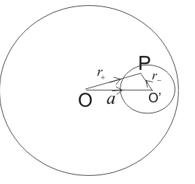From Gauss's law we know that electric field strength inside a uniformly charged sphere is
$\mathbf{E_{sphere}}=\frac{\rho}{3 \epsilon_0}\mathbf{r}$

So electric field at any point P having position vector $\mathbf{OP}=\mathbf{r}_{+}$ is given by
$\mathbf{E_{sphere}}=\frac{\rho}{3 \epsilon_0}\mathbf{r_{+}}$

We now treat the cavity as the sphere of charge density $-\rho$. Then electric field due to cavity at point P acting along O'P is
$\mathbf{E}_{\mathrm{cavity}}=-\frac{\rho}{3\varepsilon_0}\mathbf{r}_-$
Now net electric field at p is the vector sum of $\mathbf{E}_{sphere}$ and $\mathbf{E}_{cavity}$
Thus
$\mathbf{E}=\frac{\rho}{3\varepsilon_0}\mathbf{r}_+-\frac{\rho}{3\varepsilon_0}\mathbf{r}_-=\frac{\rho}{3\varepsilon_0}(\boldsymbol{r}_+ - \boldsymbol{r}_-)=\frac{\rho}{3\varepsilon_0}\mathbf{a}$
As seen from the expression, the electric field found is uniform and constant.

Question 9
An electric dipole is placed at a distance x from a infinitely long rod of linear charge density λ.
1. Find the net amount of force acting on the dipole.
2. Assuming that dipole is fixed at its centre find its time period of oscillations if the dipole is slightly rotated about its equilibrium position.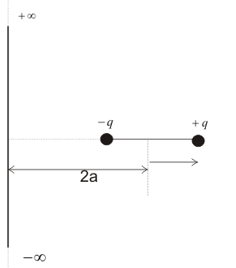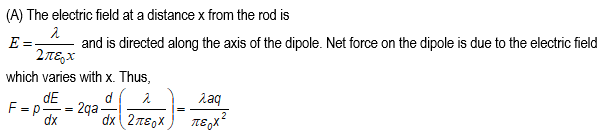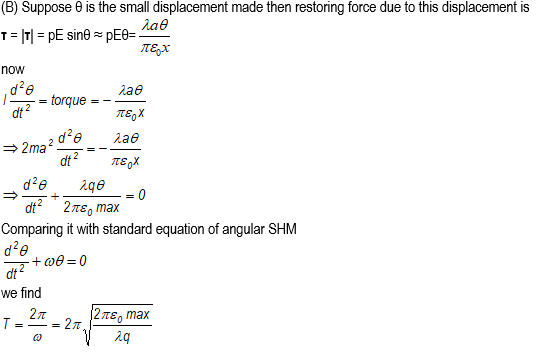Question 10
An electric charge Q is uniformly distributed over the surface of a sphere of radius R. Show that the force on a small charge element dq is radially outwards and is given by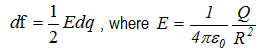is the electric field at the surface of the sphere.

Surface charge density of the sphere is
$\sigma=\frac{Q}{4\pi R^2}$
Consider the figure given below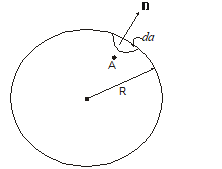where A is any point inside the sphere close to an area element da. Let dq be the amount of charge on this area element da. This charge dq will produce an electric field at point A that is approximately the one due to a uniformly charged infinitesimal charged plate. So,
$\mathbf{E}_{A'}=-\frac{\sigma}{2\epsilon_0}\mathbf{n }$
where n is the unit vector normal to da in the outward direction.
We know that the electric field is zero inside the sphere. So, total field at point A should also be equal to zero. If is the electric field at A due to all the charges on the spherical surface except da then,
$E_A=E_{A'}+E_{A''}=0$
or
$\mathbf{E}_{A''}=\frac{\sigma}{2\epsilon_0}\mathbf{n}=\frac{Q}{8\pi\varepsilon_0R^2}\boldsymbol{n}$
Since point A is close to da, $E^{"}$ may be considered as the field strength at da due to all charges on the spherical surface. Hence force acting on da is
$d\mathbf{F}=\mathbf{E}_{A''}dq=\frac{1}{2}Edq\mathbf{n}$
Where E is the field strength on the spherical surface

Question 11
A thin fixed ring of radius R has a positive charge of +q C uniformly distributed over it. A particle of mass m and charge –q is placed on axis at a distance x from the centre of the ring. Show that the motion of negatively charged particle is approximately simple harmonic.

Consider two small and equal elements of charge $\Delta q$ on the opposite sides of a diameter of a ring as shown below in the figure.
Let P is the point on the axis of the ring at a distance x from the centre. The force on charge -q placed at point P would be
$\Delta F = \frac {1}{4 \pi \epsilon _0} \frac {-q \Delta q}{AP^2}$
which is directed from P to A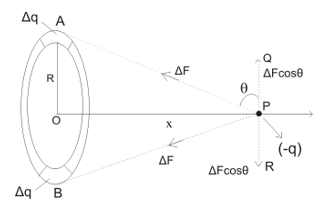This force $\Delta F$ can be resolved into two components $\Delta F \sin \theta$ along PO and $\Delta F \cos \theta$ along PQ. Similarly element of charge $\Delta q$ at B exerts a force on negative charge -q at P equal in magnitude to $\Delta F$ but directed along PB. This force$\Delta F$ can also be resolved into two components $\Delta F \sin \theta$ along PO and $\Delta F \cos \theta$ along PR which cancels out $\Delta F \cos \theta$ along PQ. Summing over all such elements over the ring , the net force along PO would be
$F=\sum\Delta F s i n\theta=-\frac{1}{4\pi\epsilon_0}\frac{q^2sin\theta}{{(AP)}^2}$
From triangle AOP
$\sin \theta=\frac {OP}{AP}$
Thus
$F=-\frac{q^2(OP)}{4\pi\epsilon_0{(AP)}^3}$
Now OP=x and $AP=\sqrt {x^2 + R^2}$
Or,
$F=-\frac {q^2x}{4\pi\epsilon_0 {(x^2+R^2)}^{\frac{3}{2}}}$
For R>>x , $(x^2+R^2)^{3/2} \approx R^3$
$F=-\frac {q^2x}{4\pi\epsilon_0R^3}$
Since acceleration a=F/m comparing this wit above equation we get
$a=\frac{F}{m}=-\omega^2x$
Where $\omega=\left[\frac{q^2}{4\pi\varepsilon_0mR^3}\right]^{1/2}$
Since acceleration is proportional to negative of displacement x motion of charge would be simple harmonic motion

Question 12
Consider the figure given below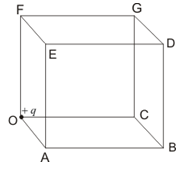A positive charge +q is placed at corner of the cube. Find the electric flux through the right face BCGDB of the cube.

Think of this cube as one of 8 surrounding the charge. Each of the 24 squares which make up the surface of this larger cube gets the same flux as every other one, so $\int _{one face} E.da=\frac {1}{24} \int _{whole cube} E.da$
From Gauss's law $\int E.da=\frac {q}{\epsilon _0}$
Hence electric flux through face BCDGB is
$Flux = \frac {q}{24 \epsilon _0}$

Question 13
Consider a sphere of radius r having charge q C distributed uniformly over the sphere. This sphere is now covered with a hollow conducting sphere of radius R>r.
1. Find the electric field at point P away from the centre O of the sphere such that r<OP<R.
2. Find the surface charge density on the outer surface of the hollow sphere if charge q’ C is placed on the hollow sphere.

(a)Consider the figure given below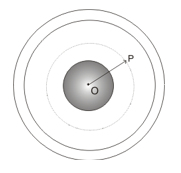We need to find the field at the point P. First, we draw the concentric spherical Gaussian surface through point P. Field at all points on the Gaussian surface would be equal in magnitude and direction of the field would be in radial outwards direction. Now flux through the surface is
$\phi=\oint{\mathrm{E.ds}=E\oint d s}=E(4\pi{r}^2)$
Where OP=r
From Gauss s Law
$\oint{\mathrm{E.ds}} = \frac {q_{enc}}{\epsilon _0}$
Since $q_{enc} = q$ the charge enclosed inside the Gaussian surface
$E \times 4 \pi r^2 = \frac {q}{\epsilon _0}$
$E=\frac{q}{4\pi\epsilon_0 r^2}$
(b) For finding the charge on the outer surface of the hollow sphere we draw Gaussian surface through the material of the hollow sphere as shown below in the figure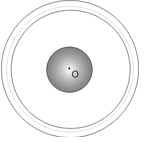We know that electric field inside the conductor is zero so the flux through the Gaussian surface would also be zero. From Gauss's law we conclude that total charge enclosed in Gaussian surface is zero. Hence charge on inner surface of hollow sphere is q C. Hence total charge on outer surface of hollow sphere is
$q_{outer}=(q^{'}+q)$ C

Question 14
1. Find the electric field inside the uniformly charged sphere of radius R and volume charge density ρ using Gauss’s law.
2. Use Gauss’s law to find the electric field outside, at a point on the surface and at any point inside a spherical shell of radius R, carrying a uniform surface charge density σ.

(a) We need to find the electric field inside the uniformly charged sphere of radius R and volume charge density $\rho$ using Gauss's law. In this case, Gaussian surface is a spherical surface, whose centre is at O and radius equal to r where r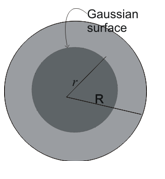$E\cdot4\pi r^2=\frac{q'}{\epsilon_0}$
or
$E=\frac{1}{4\pi\epsilon_0}\frac{q'}{r^2}$
Now
$q'=\frac{q}{4/3(\pi R^3)}\times\frac{4}{3}\pi r^3=\frac{qr^3}{R^3}$
From this
$E=\frac{1}{4\pi\epsilon_0}\frac{qr}{R^3}$
$\because q=\frac{4}{3}\pi R^3\rho$
$E=\frac{\rho r}{3\epsilon_0}$
Thus, as we get near to the centre, the intensity falls from maximum to zero at the centre. Hence intensity at point P inside a charged sphere, in which the charge is uniformly distributed, is directly proportional to distance of point P from the centre of the sphere as shown below in the figure.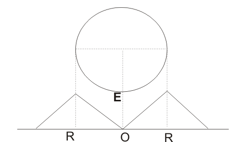(b)Now we need to use Gauss's law to find the electric field outside, at a point on the surface and at any point inside a spherical shell of radius R, carrying a uniform surface charge density $\sigma$. Now total charge on the shell is
$q=4 \pi R^2 \sigma$
For solving the problem consider the figure given below which shows the Gaussian surface (dotted surface) for points inside and outside the shell.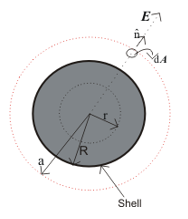(1) For points outside the shell:-
Draw a sphere of radius a concentric with the shell to represent the Gaussian surface. Total electric flux over a spherical surface of radius a is
$\int_{s}{E\cdot d}A=|E|\int_{s} d A=|E|4\pi a^2$
From Gauss's law flux
$\int_{s}{E\cdot d}A=\frac{q}{\epsilon_0}$
$\Rightarrow|E|4\pi a^2=\frac{q}{\epsilon_0}$
$\mathbf{E}=\frac{q}{4\pi\epsilon_0a^2}\hat{n}$
For a>R. Thus above equation gives the electric field intensity at a point outside the charged spherical shell. In terms of surface charge density field intensity can be calculated by putting $q=4 \pi R^2 \sigma$ in above equation and the result obtained is
$\mathbf{E}=\frac{\sigma R^2}{\varepsilon_0a^2}\hat{\mathbf{n}}$

(2) At a point on the surface of the shell
For point on the surface of the shell a=R
$E=\frac{\sigma a^2}{\epsilon_0a^2}=\frac{\sigma}{\epsilon_0}$

(3) At a point inside the shell
In this case r $\int_{s}{E\cdot d}A=\frac{q}{\epsilon_0}$
$|E|4\pi a^2=0$
E=0
inside the spherical shell having uniform surface charge density.

Question 15
(a) Show that the normal component of electrostatic field has a discontinuity from one side of a charged surface to another given by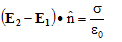Where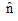a unit vector is normal to the surface at a point and σ is the surface charge density at that point. (The direction of is from side 1 to side 2.)
(b) Show that the tangential component of electrostatic field is continuous from one side of a charged surface to another.

(a) We know that statement of Gauss's law in integral form is
$\oint E.dS= \frac {Q_{enc}}{\epsilon_0}$
Now we have to show that that the electric field always undergoes a discontinuity when surface charge $\sigma$ is crossed.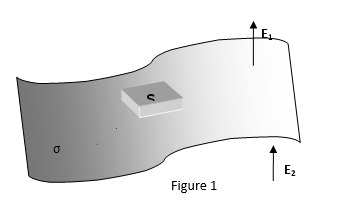Let's draw a wafer-thin Gaussian pillbox, extending just over the edge in each direction. Apply Gauss's law to Gaussian pillbox S of cross-sectional area A whose two ends are parallel to the interface. The ends of the box can be made arbitrarily close together. In this limit, the flux of the electric field out of the sides of the box is obviously negligible, and the only contribution to the flux comes from the two ends. Thus
$\oint \mathbf{E}.d\boldsymbol{S}= ( \mathbf{E_1 -E_2}).\mathbf{n} A$
where $E_1$ is the component of electric field normal to the interface immediately above the surface and $E_2$ is the component of electric field normal to the interface immediately below the surface The charge enclosed by the pill-box is simply $\sigma A$, where $\sigma$ is the sheet charge density on the interface . Thus, Gauss' law yields
$( \mathbf{E_1 -E_2}).\mathbf{n} =\frac {\sigma}{\epsilon _0}$

Where $\mathbf{n}$ a unit vector is normal to the surface at a point and $\sigma$ is the surface charge density at that point. (The direction of $\mathbf{n}$ is from side 1 to side 2.) Thus that the normal component of electrostatic field has a discontinuity from one side of a charged surface to another i.e., the presence of a charge sheet on an interface causes a discontinuity in the perpendicular component of the electric field.
Just outside a conductor, the electric field is $\frac {\sigma}{\epsilon _0}\mathbf{n}$

(b) Now we have to show that tangential component of E, by contrast, is always continuous. For this consider the figure shown below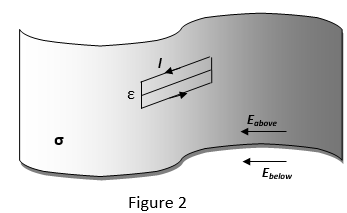In the above figure consider the thin rectangular loop of length l and width e -> 0
Now we consider one of the Maxwell's equation known Faraday's law in integral form which is
$\oint _c \mathbf{E}.d\mathbf{l}=- \frac {d}{dt}\int _s \mathbf{B}.\mathbf{n} da$

Here right-hand side leads to A(B)normal , but A -> 0 as sides of the loop are very short nearly approaching zero i.e., e -> 0 , hence contribution due to magnetic effects vanishes. We thus have,
$\oint _c \mathbf{E}.d\mathbf{l}=0$
Now if we apply this integral to thin rectangular loop as shown in figure 2 then the dominant contribution to the loop integral comes from the long sides because he length of the short sides is assumed to be arbitrarily small which ends up giving nothing. Thus, we have
$E_{above} - E_{below}=0$
or
$E_{above} =E_{below}$

Where, $E_{above}$ and $E_{below}$ are tangential components of electric field thus there can be no discontinuity in the parallel component of the electric field across an interface.

Question 16
Consider a cylinder as given below in the figure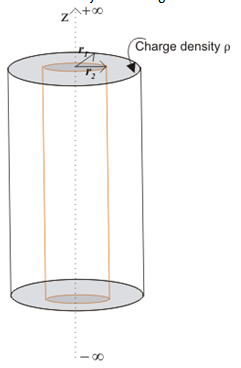Volume between radius r1 and r2 contains uniform charge density ρ C/m3. Use Gauss’s law to find electric field in all regions.

Consider the figure given in question.
(a) For region $0 < r < r_1$ from Gauss s law we have
$\oint E.dS= \frac {Q_{enc}}{\epsilon_0}$
or $\frac {q_{enc}}{\epsilon_0}=E(2 \pi r L)$
where L is the length of cylindrical segment under consideration. Since charge enclosed in this region is
$q_{enc}=0$ therefore from gauss's law E=0 in this region.

(b) For region $r_1 \leq r \leq r_2$
from gauss's law for cylinders we have
$\frac {q_{enc}}{\epsilon_0}=E(2 \pi r L)$
Consider a Gaussian cylinder of radius r inside the cylindrical shell such that $r_1=r=r_2$. Volume of cylinder of radius r and length L is given by
$V= \pi r^2L$
Thus charge enclosed is
$q_{enc}=\rho \pi [r^2-(r_1^2]L$
using gauss's law
$\frac{\rho\pi\left(r^2-r_1^2\right)L}{\epsilon_0}=E(2\pi rL)$
or
$E=\frac{\rho\left(r^2-r_1^2\right)}{2r\epsilon_0}$

(c) For $r>r_2$
Gaussian surface here is a cylinder of radius $r > r_2$. Now charge enclosed inside the Gaussian surface is
$q_{enc}= \rho \pi [(r_2)^2-(r_1)^2]$
Form Gauss's law ,
$\frac {q_{enc}}{\epsilon_0}=E(2 \pi r L)$
$\frac{\rho\pi\left({r_2}^2-r_1^2\right)L}{\epsilon_0}=E(2\pi rL)$
or
$E=\frac{\rho\left(r_2^2-r_1^2\right)}{2r\epsilon_0}$